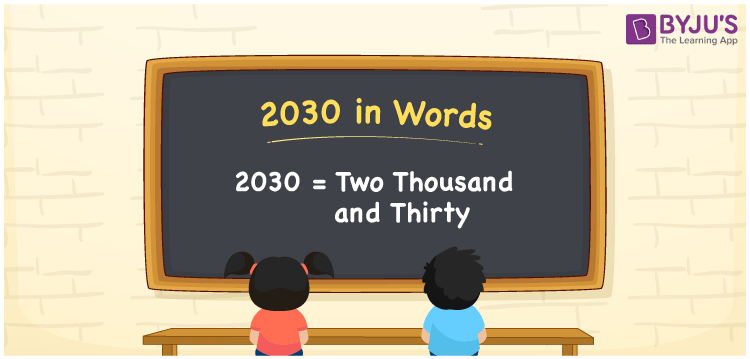# 2030 in Words

The spelling of 2030 in English words is two thousand and thirty. The number has 4 digits and it is a cardinal number. For example, the cost of a chair is Rs. 2030. This can also be written as “The cost of a chair is Rs. Two thousand and thirty”. Now, we are going to learn the process of writing the number 2030 in words using the place value system in detail.

 2030 in Words: Two Thousand and Thirty. Two Thousand and Thirty in Numerical Form: 2030.

## 2030 in English Words## How to Write 2030 in Words?

The following is the place value table for the number 2030.

 Thousands Hundreds Tens Ones 2 0 3 0

The expanded form of 2030 is as follows:

= 2 × Thousand + 0 × Hundred + 3 × Ten + 0 × One

= 2 × 1000 + 0 × 100 + 3 × 10 + 0 × 1

= 2000 + 30

= 2030

= Two thousand and thirty

Hence, 2030 in words is two thousand and thirty.

2030 in words – Two thousand and thirty

Is 2030 an odd number? – No

Is 2030 an even number? – Yes

Is 2030 a perfect square number? – No

Is 2030 a perfect cube number? – No

Is 2030 a prime number? – No

Is 2030 a composite number? – Yes

## Frequently Asked Questions on 2030 in Words

Q1

### How to spell 2030?

2030 in words is two thousand and thirty.

Q2

### Simplify 2000 + 30, and express it in words.

Simplifying 2000 + 30, we get 2030. Hence, 2030 in words is two thousand and thirty.

Q3

### Is 2030 an even number?

Yes, 2030 is an even number.